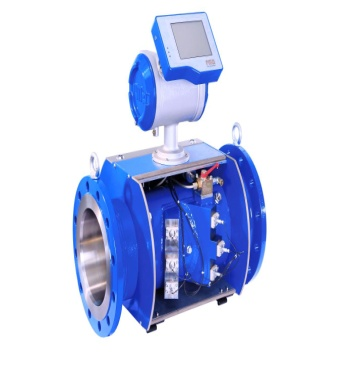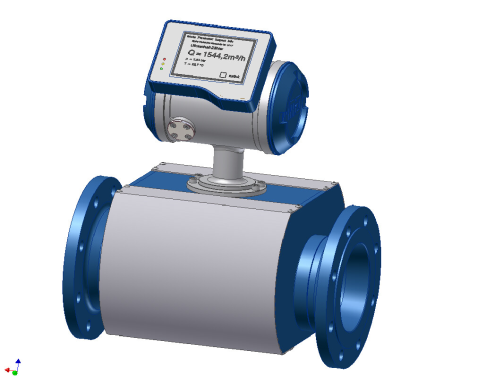# CEDCO / Products / Ultrasonic Flow Meter

Ultrasonic Flow Meter

Flow measurement is the quantification of bulk fluid movement. Flow can be measured in a variety of ways. Positive-displacement flow meters accumulate a fixed volume of fluid and then count the number of times the volume is filled to measure flow. Other flow measurement methods rely on forces produced by the flowing stream as it overcomes a known constriction, to indirectly calculate flow. Flow may be measured by measuring the velocity of fluid over a known area.
Both gas and liquid flow can be measured in volumetric or mass flow rates, such as liters per second or kilograms per second. These measurements can be converted between one another if the material's density is known. The density for a liquid is almost independent of the liquid conditions; however, this is not the case for gas, the density of which depends greatly upon pressure, temperature and to a lesser extent, the gas composition.
When gases or liquids are transferred for their energy content, such as the sale of natural gas, the flow rate may also be expressed in terms of energy flow, such as GJ/hour or BTU/day. The energy flow rate is the volume flow rate multiplied by the energy content per unit volume or mass flow rate multiplied by the energy content per unit mass. Where accurate energy comes to the time of the legit flow rate is desired, most flow meters will be used to calculate the volume or mass flow rate which is then adjusted to the energy flow rate by the use of a flow computer.

• How is the ideal gas meter?
• Exactly and reliably measured values
• Calculation of base volume without additionally equipment
• Large measuring range
• No moving parts,no abrasion and maintenance-free
• No pressure drop
• Possibilities for data transfer and remote control
• Reasonable costs by buying and in the operation